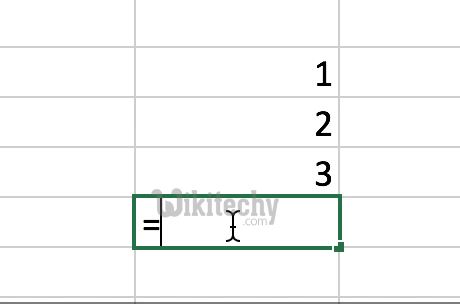# pig tutorial - apache pig tutorial - Apache Pig SUM() Function - pig latin - apache pig - pig hadoop

## What is SUM() Function in Apache Pig ?

• The SUM() function used in Apache Pig is used to get the total of the numeric values of a column in a single-column bag.
• The SUM() function ignores the NULL values while computing the total
• The SUM() Function will requires a preceding GROUP ALL statement for global sums and the GROUP BY statement for group sums.
• The SUM() function can add individual values, cell references and the ranges.
• The SUM() Function will adds all the numbers which are given in a column.learn pig tutorial - apache pig sum function - pig example

## wikitechy_employee.txt

• We have loaded the file into Pig with the relation name called employee_data which is given below:

## Calculating the Sum of All GPA

• We need to group the relation name employee_data by using the Group All operator, and we need to store the result in the relation name employee_group which is given below:
• It will produce a relation for calculating the sum of all gpa which is given below:
• Now, we will need to calculate the global sum of the pages which is typed daily.